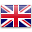# age 10 - 12

Sort by:
##### 4.1 探索電路 Exploring Circuit

STEM lessons + blocks

Students will gain understanding of how electricity is...##### 4.10 比率 Ratio

STEM lessons + blocks

Students will work with collections of physical objects,...##### 4.2 地球和軌跡 Earth and Orbit

STEM lessons + blocks

Students will gain understanding of what an orbit...##### 4.3 黑夜與白晝 Night and Day

STEM lessons + blocks

Students will gain understanding of how the Earth...##### 4.4 摩斯密碼警報 Morse Code Alert

STEM lessons + blocks

Students will extend their knowledge of Morse Code,...##### 4.5 密碼與模式 Password and Patterns

STEM lessons + blocks

In the first session, students will...##### 4.6 牛頓第三運動定律 Every Action ....

STEM lessons + blocks

Students will gain understanding of Newton’s Third Law...##### 4.7 探索遺傳 Exploring Heredity

STEM lessons + blocks

Students will work collaboratively to investigate heredity and...##### 4.8 遺傳與變異 Grey or Ginger Kittens

STEM lessons + blocks

Students will investigate how genetic variation of traits...##### 4.9 飲水機監控系統 Water Dispenser

STEM lessons + blocks

Students will gain understanding of the positive and...